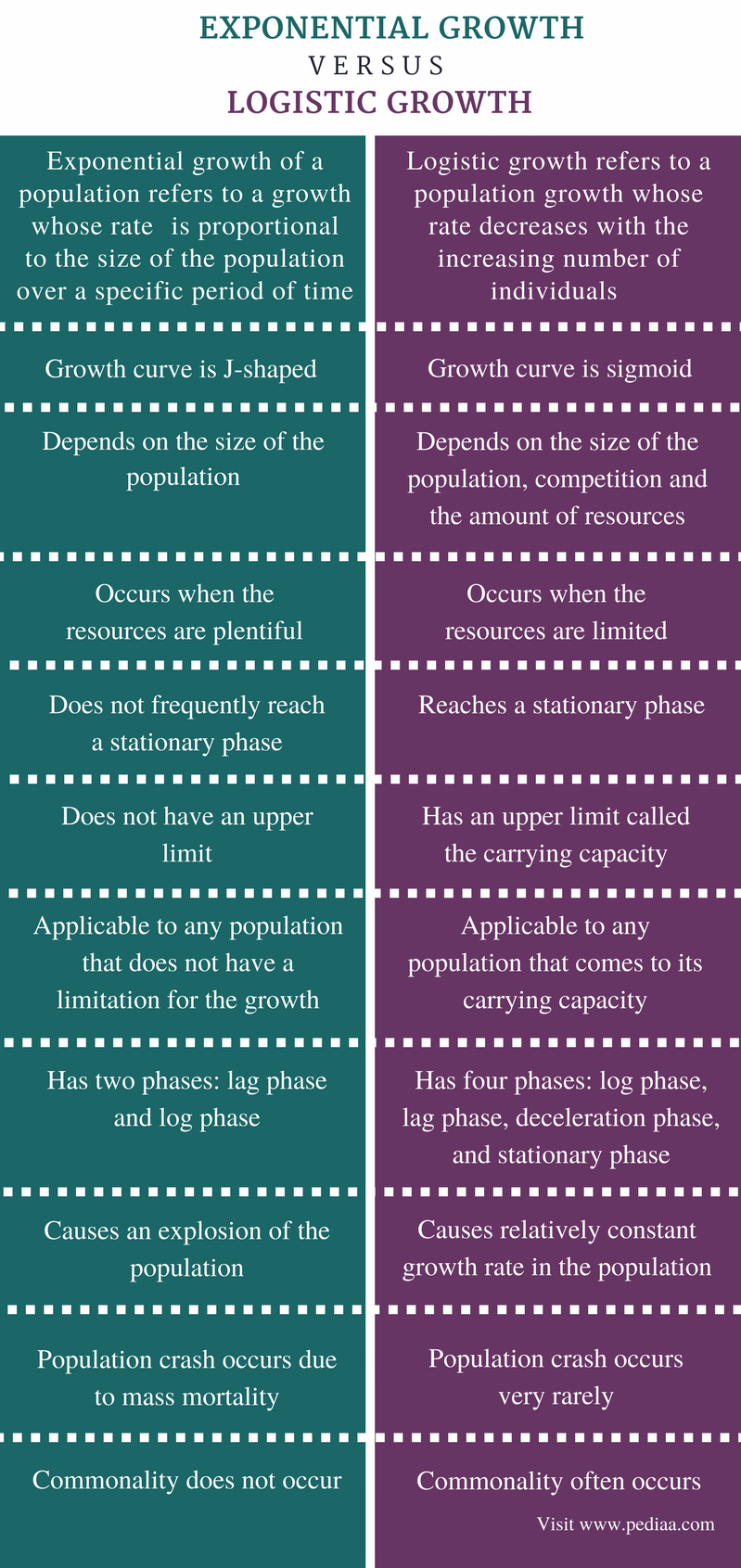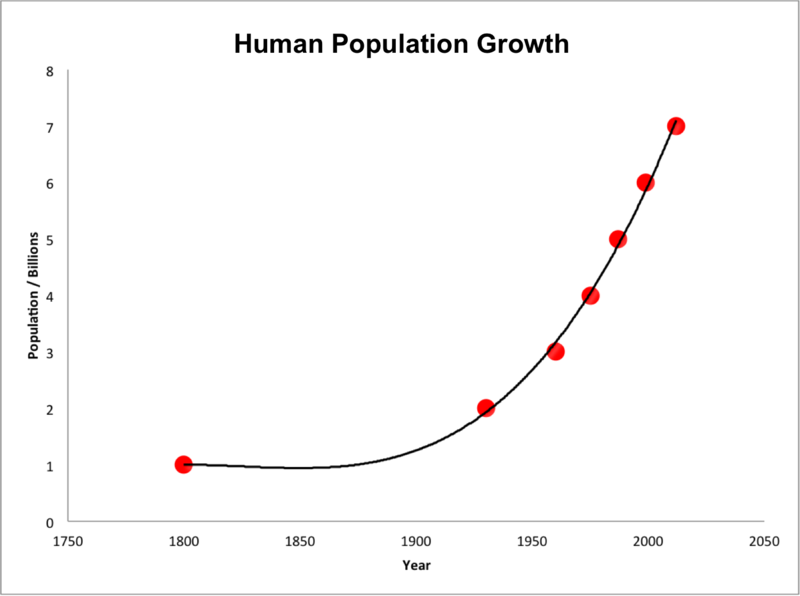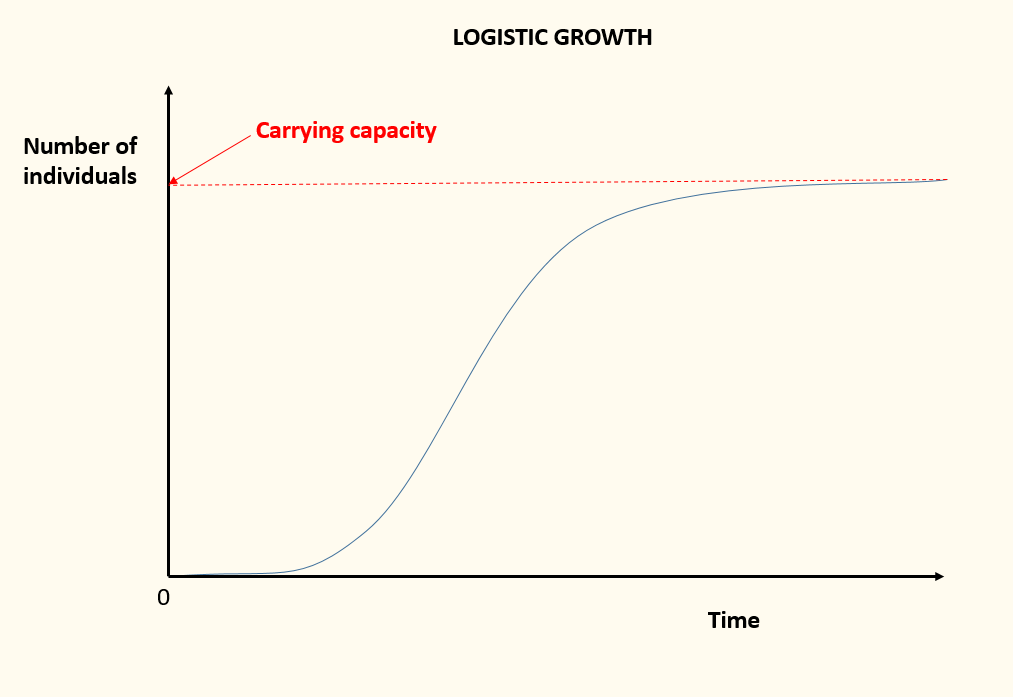# Difference Between Exponential and Logistic Growth

## Main Difference – Exponential Growth vs Logistic Growth

Exponential growth and logistic growth are two terms used to describe the growth of populations. The increase of the size of the population over a specific time period is referred to as the growth of the population. The growth rate of the population refers to the change in the number of individuals in a particular population over time. The main difference between exponential and logistic growth is that exponential growth occurs when the resources are plentiful whereas logistic growth occurs when the resources are limited. The exponential growth is proportional to the size of the population. It is influenced by the rate of birth and the rate of death. The logistic growth is influenced by the size of the population, competition, and limited resources.

### Key Areas Covered

1. What is Exponential Growth
– Definition, Characteristics, Examples
2. What is Logistic Growth
– Definition, Characteristics, Examples
3. What are the Similarities Between Exponential Growth and Logistic Growth
– Outline of Common Features
4. What is the Difference Between Exponential Growth and Logistic Growth
– Comparison of Key Differences

Key Terms: Carrying Capacity, Competition, Doubling Time, Exponential Growth, Logistic Growth, Population Size, Rate of Birth, Rate of Death, Resources## What is Exponential Growth

The exponential growth refers to a growth of a population whose rate is proportional to the size of the population over a specific period of time. The size of the population depends on the rate of birth and the rate of death. The exponential growth occurs when plentiful of resources are available for the individuals in the population. It results in a J-shaped curve when the number of entities is plotted against time. At the beginning, the size of the population is small. With time, the size of the population increases. The growth rate rapidly increases along with the increasing size of the population. The exponential growth shows a fixed percentage rate of increase over time. The doubling time refers to the period of time required to double the number of in a particular population.Figure 1: Exponential Growth of Human Population

The most precise example of exponential growth is the growth of the human population. The increase in the number of microorganisms in a culture until the essential nutrients in the culture become limited is another example of exponential growth. The spread of a virus if no artificial immunization is available is also an example of exponential growth. The exponential growth is shown in figure 1

## What is Logistic Growth

The logistic growth refers to a population growth whose rate decreases with the increasing number of individuals and it becomes zero when the population becomes its maximum. When the food supply and space become limited, a competition arises among individuals in the population for the resources. Therefore, the rate of growth does not only depend on the size of the population. The rate of birth and the rate of death depend on the ability to grab resources in the environment. Hence, the size of the population does not exceed the carrying capacity of the environment. The carrying capacity refers to the maximum population size the environment can sustain. When the growth of the population reaches the carrying capacity of the environment, the rate of the growth decreases.Figure 2: Logistic Growth Curve

Since it is more realistic than exponential growth model, the logistic growth model can be applied to the most populations on the earth. The logistic growth is a sigmoid curve when the number of entities is plotted against time. The logistic growth is shown in figure 2

## Similarities Between Exponential and Logistic Growth

• Both exponential growth and logistic growth describe the growth of a population.
• Both exponential growth and logistic growth depend on the size of the population.

## Difference Between Exponential and Logistic Growth

### Definition

Exponential Growth: Exponential growth of a population refers to a growth whose rate is proportional to the size of the population over a specific period of time.

Logistic Growth: The Logistic growth refers to a population growth whose rate decreases with the increasing number of individuals and it becomes zero when the population becomes its maximum.

### Growth Curve

Exponential Growth: The growth curve of the exponential growth is J-shaped.

Logistic Growth: The growth curve of the logistic growth is sigmoid.

### Factors Affecting Growth

Exponential Growth: The exponential growth depends on the size of the population.

Logistic Growth: The logistic growth depends on the size of the population, competition and the amount of resources.

### Resources

Exponential Growth: The exponential growth occurs when the resources are plentiful.

Logistic Growth: The logistic growth occurs when the resources are limited.

### Stationary Phase

Exponential Growth: The exponential growth does not frequently reach a stationary phase.

Logistic Growth: The logistic growth reaches a stationary phase.

### Upper Limit

Exponential Growth: The exponential growth lacks any upper limit.

Logistic Growth: The logistic growth consists of an upper limit called the carrying capacity.

### Applicable to

Exponential Growth: The exponential growth is applicable to any population that does not have a limitation for the growth.

Logistic Growth: The logistic growth is applicable to any population that comes to its carrying capacity.

### Phases

Exponential Growth: The exponential growth has two phases: lag phase and log phase.

Logistic Growth: The logistic growth has four phases: log phase, lag phase, deceleration phase, and stationary phase.

### Cause

Exponential Growth: The exponential growth causes an explosion of the population.

Logistic Growth: The logistic growth causes relatively constant growth rate in the population.

### Population Crash

Exponential Growth: The population crash occurs due to the mass mortality during the exponential growth.

Logistic Growth: The population crash occurs very rarely during the logistic growth.

### Commonality

Exponential Growth: Commonality does not occur often in the exponential growth.

Logistic Growth: Commonality occurs very often in the logistic growth.

### Conclusion

Exponential growth and logistic growth are two types of growth of populations. The exponential growth is the increase in the population size when plentiful of resources are available. The logistic growth occurs when the increase in the size of the population is influenced by the limited resources in the environment. The main difference between exponential growth and logistic growth is the factors that affect each type of growth.

##### Reference:

1.“Exponential Growth: Definition & Examples.” Study.com, Available here. Accessed 18 Sept. 2017.
2.“Logistic Growth” Personal Kenyon.edu, Available here. Accessed 18 Sept. 2017.

##### Image Courtesy:

1. “Human population growth from 1800 to 2000″By User:Clevercapybara – created by User:Clevercapybara (CC BY 3.0) via Commons Wikimedia### About the Author: Lakna

Lakna, a graduate in Molecular Biology & Biochemistry, is a Molecular Biologist and has a broad and keen interest in the discovery of nature related things